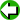# Inductive Proof vs. Deductive Proof

Summary: Using a Squares Table to understand Inductive vs. Deductive proof.

Have a squares table on the board before class begins.

Explain definition of an inductive proof: An inductive proof is where mathematical statements are justified by examples. We look at a data set and based on a characteristic of the data set believe that the characteristic is proven by the fact that it is always present in our data set. Our inductive proof is made stronger by adding elements to our data set that continue to have the characteristic. If we ever find an element of our data set that does not have the characteristic our inductive proof is null and void!

Ask the students if they see anything interesting about the numbers in the squares table. Hopefully someone will say, ‘There are no 2’s, 3’s, 7’s, or 8’s’ in the ones place.

Then say, ‘So, our characteristic is that a whole number squared will not have a 2, 3, 7, or 8 in its ones’ digit place. None of the elements in our data set have a 2, 3, 7, or 8 in their ones’ digit place so we have an inductive proof that a whole number squared will not have a 2, 3, 7, or 8 in its ones’ place. We can add elements to our table to see if it’s true for them also. (Do so). And you see that it is. Incidentally, by adding elements we have strengthened our inductive proof. But we don’t absolutely know that our proof is true because if we keep adding elements maybe we would eventually find one that did have a 2, 3, 7, or 8 in its ones’ digit place. And if we found just one it would destroy our proof. You can see that an inductive proof is weaker than we would like.’

Continuing, ‘Now let’s look at deductive proofs. A deductive proof if where we have one or more indisputable facts that necessarily mean that something is true. This is a ‘strong’ proof. If the facts we use to support the truth of our characteristic are true, then the characteristic must also be true. For example, if I tell you that if a person has a million dollars then they are a millionaire, and then tell you that Susan has a million dollars, we could form a deductive proof and claim that Susan is a millionaire. Now if it is really true that Susan has a million dollars, it is also true that she is a millionaire. No getting around it. This is the kind of proof we want to have in mathematics. But deductive proofs are harder to do than inductive proofs.’

‘I now invite the class to think up a deductive proof to show that whole numbers squared cannot have a 2, 3, 7, or 8 in their ones’ digit place.’

See how they do.

0x0=0 1x1=1 2x2=4 3x3=9 4x4=16 5x5=25 6x6=36 7x7=49 8x8=64 9x9=81 these are the only possibilities for whole numbers squared one’s digit to be: 0, 1, 4, 5, 6, or 9. Thus they cannot be 2, 3, 7, or 8.
Submitted by: Randall Hudson Rlostsoul53@email-removedBack to our Geometry Lesson Plans

107.161.24.116